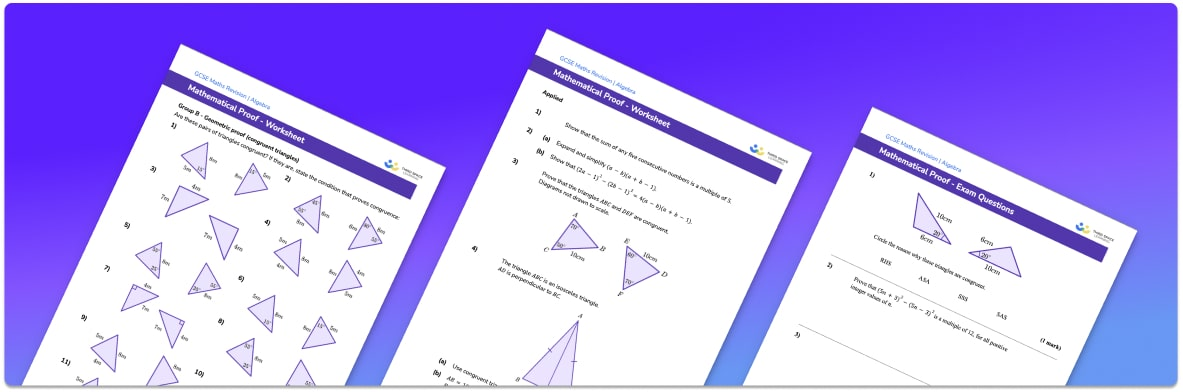# Mathematical proof worksheet• Section 1 of the mathematical proof  worksheet contains 36 skills-based mathematical proof  questions, in 3 groups to support differentiation
• Section 2 contains 5 applied mathematical proof  questions with a mix of worded problems and deeper problem solving questions
• Section 3 contains 4 foundation and higher level GCSE exam style questions on mathematical proof
• Answers and a mark scheme for all mathematical proof questions are provided
• Questions follow variation theory with plenty of opportunities for students to work independently at their own level
• All questions created by fully qualified expert secondary maths teachers
• Suitable for GCSE maths revision for AQA, OCR and Edexcel exam boards

• This field is for validation purposes and should be left unchanged.

You can unsubscribe at any time (each email we send will contain an easy way to unsubscribe). To find out more about how we use your data, see our privacy policy.

### Mathematical proof at a glance

A mathematical proof is a logical sequence of mathematical statements that proves that something is always true. For example, we could use algebra to prove that the sum of two consecutive odd numbers is always a multiple of 4 or we could use circle theorems to prove a statement about angles.

Algebraic proofs involve working from a starting point, which we write on the left hand side. They often require the expansion of brackets followed by the manipulating/simplifying/ factorising of the algebraic expression, which we write in a logical step by step way on the right hand side. We continue until a conclusion can be made about the final expression. When working on algebraic proofs, we generally use 2n when we want to represent an even number (integer) and 2n+1 to represent an odd integer.

Geometrical proofs could involve using angle facts or circle theorems to prove statements about angles or using our knowledge of vectors to prove geometric statements. Sometimes, problems might involve using our knowledge of geometry and algebra together to form a proof.

We could also be asked to prove something by counterexample. This means finding an example which doesn’t work for the given statement, therefore proving it is not alway true. For example, if you are given the statement that if you add 1 to a multiple of 4 you always get a prime number, you could prove this is not true by finding an example where it doesn’t work.

Mathematical proof is studied further in A Level maths, and other methods of proof, such as proof by induction and proof by contradiction, are introduced.

Looking forward, students can then progress to additional proofs in maths worksheets or more algebra worksheets, for example a sequences worksheet, simultaneous equations worksheet or straight line graphs worksheet.For more teaching and learning support on Algebra our GCSE maths lessons provide step by step support for all GCSE maths concepts.

## Do you have KS4 students who need more focused attention to succeed at GCSE?There will be students in your class who require individual attention to help them succeed in their maths GCSEs. In a class of 30, it’s not always easy to provide.

Help your students feel confident with exam-style questions and the strategies they’ll need to answer them correctly with our dedicated GCSE maths revision programme.

Lessons are selected to provide support where each student needs it most, and specially-trained GCSE maths tutors adapt the pitch and pace of each lesson. This ensures a personalised revision programme that raises grades and boosts confidence.

Find out more